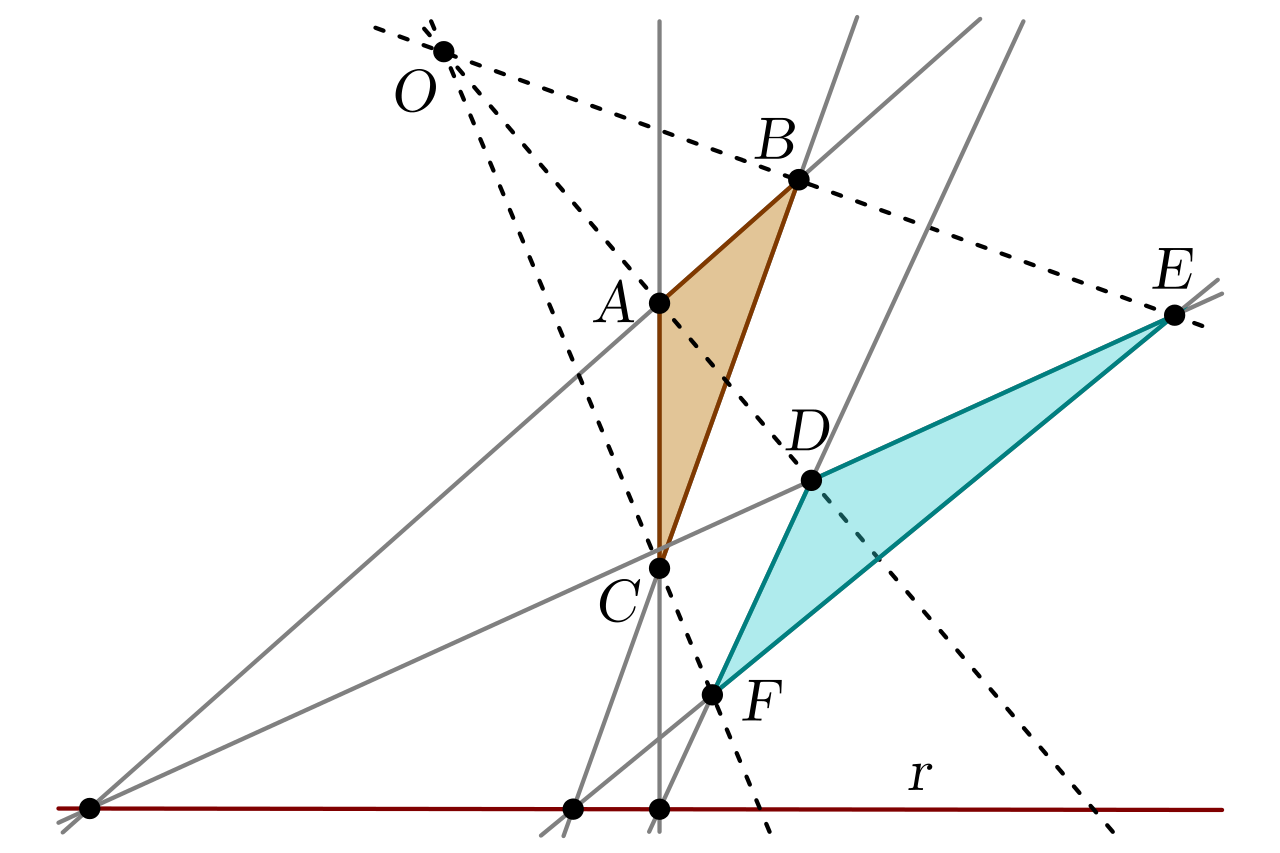# Geometry

Join us here and learn all about Geometry and download tools to help kids better understand the principles.

## What is Geometry?

Geometry allows us to discover patterns, find areas, volumes; lengths and angles, and improve our understanding of the world we live in. Geometry is all about shapes and their properties. Geometry is one of the first models of math. The word geometry in the Greek dialect interprets the words for "Earth" and "Measure". The Egyptians were one of the first to utilize geometry. The Egyptians utilized right triangles to quantify and appraise land. In our times, geometry is utilized in the fields, designing, structural engineering, medication, drafting, space science, and geology.

Geometry can be divided into:

Plane Geometry A flat surface that extends into infinity in all directions is known as a Plane.

Solid Geometry The branch of math that comprises three-dimensional figures and surfaces.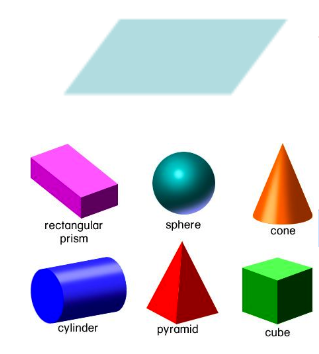## Point, Line, Plane and Solid

A Point is an exact location in space. A point has no size.

Line extends indefinitely in a single dimension.

Plane exists in two dimensions.

Solid is a 3-dimensional figure that has width, depth and height.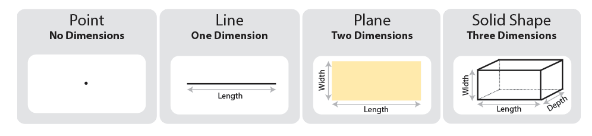## Plane Geometry

Plane Geometry is about shapes on a flat surface (like on an piece of paper). The plane has two dimensions: length and width. Keep in mind, that the plane is infinite in size; the length and width can't be measured.

Polygons

A Polygon is a 2-dimensional shape made of straight lines. Triangles and Rectangles are polygons.

The Circle

A Circle is a perfectly round shape: a line that is curved so that its ends meet and every point on the line is the same distance from the center.

Symbols

Symbols help save time and space for mathematicians. Below is a short list of common symbols used in Geometry: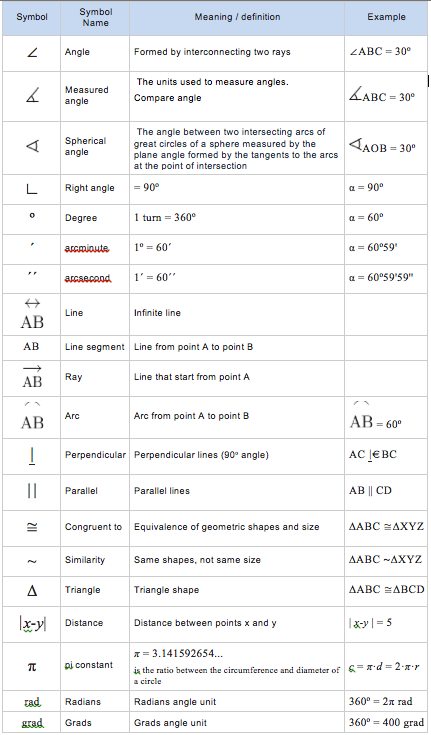Angles

The space inside of two lines or three or more planes veering from a typical point, or inside of two planes separating from a typical line.

Types of Angles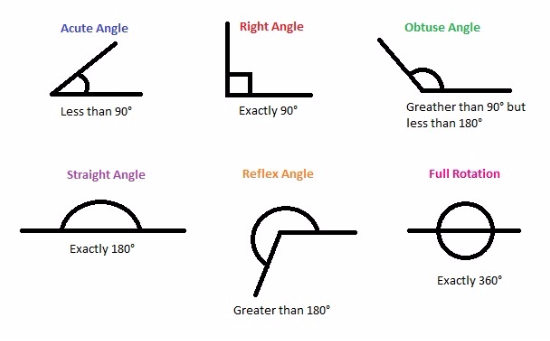Coordinates One of an arrangement of numbers that decides the position of a point. One and only one coordinate is required if the point on a line, two are needed if the point is in a plane, and three in the event that it is in space.